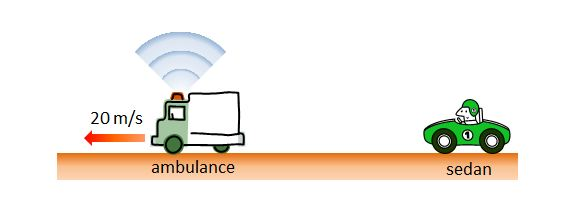# How fast is the sedan?In the above diagram, an ambulance moving at a constant speed of $20 \text{ m/s}$ generates a sound of frequency $1080 \text{ Hz}.$ Calvin is chasing the ambulance in his sedan at a constant speed. If the frequency of the sound measured by Calvin from his car is $1050 \text{ Hz},$ then what is the speed of Calvin's sedan?

The speed of sound in the air is $340 \text{ m/s}.$

×

Problem Loading...

Note Loading...

Set Loading...